# Dunn index and DB index – Cluster Validity indices | Set 1

Different performance metrics are used to evaluate different Machine Learning Algorithms. In case of classification problem, we have a variety of performance measure to evaluate how good our model is. For cluster analysis, the analogous question is how to evaluate the “goodness” of the resulting clusters?

Why do we need cluster validity indices ?

• To compare clustering algorithms.
• To compare two sets of clusters.
• To compare two clusters i.e which one is better in terms of compactness and connectedness.
• To determine whether random structure exists in the data due to noise.
•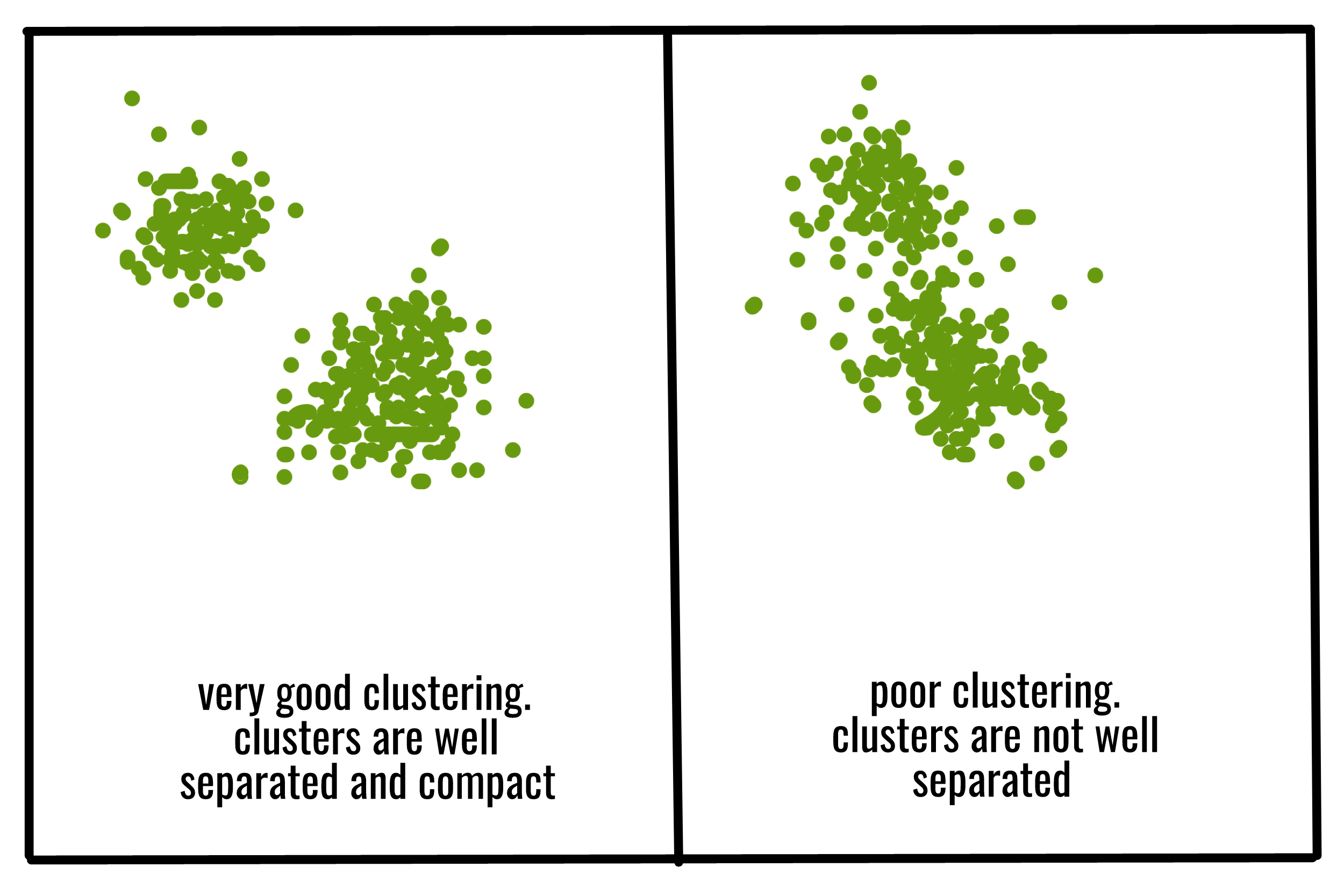Generally, cluster validity measures are categorized into 3 classes, they are –

1. Internal cluster validation : The clustering result is evaluated based on the data clustered itself (internal information) without reference to external information.
2. External cluster validation : Clustering results are evaluated based on some externally known result, such as externally provided class labels.
3. Relative cluster validation : The clustering results are evaluated by varying different parameters for the same algorithm (e.g. changing the number of clusters).

Besides the term cluster validity index, we need to know about inter-cluster distance d(a, b) between two cluster a, b and intra-cluster index D(a) of cluster a.

Inter-cluster distance d(a, b) between two clusters a and b can be –

• Single linkage distance: Closest distance between two objects belonging to a and b respectively.
• Complete linkage distance: Distance between two most remote objects belonging to a and b respectively.
• Average linkage distance: Average distance between all the objects belonging to a and b respectively.
• Centroid linkage distance: Distance between the centroid of the two clusters a and b respectively.

Intra-cluster distance D(a) of a cluster a can be –

• Complete diameter linkage distance: Distance between two farthest objects belonging to cluster a.
• Average diameter linkage distance: Average distance between all the objects belonging to cluster a.
• Centroid diameter linkage distance: Twice the average distance between all the objects and the centroid of the cluster a.

Now, let’s discuss 2 internal cluster validity indices namely Dunn index and DB index.

### Dunn index :

The Dunn index (DI) (introduced by J. C. Dunn in 1974), a metric for evaluating clustering algorithms, is an internal evaluation scheme, where the result is based on the clustered data itself. Like all other such indices, the aim of this Dunn index to identify sets of clusters that are compact, with a small variance between members of the cluster, and well separated, where the means of different clusters are sufficiently far apart, as compared to the within cluster variance.
Higher the Dunn index value, better is the clustering. The number of clusters that maximizes Dunn index is taken as the optimal number of clusters k. It also has some drawbacks. As the number of clusters and dimensionality of the data increase, the computational cost also increases.

The Dunn index for c number of clusters is defined as :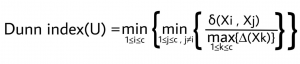where,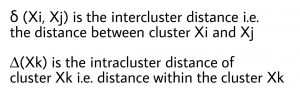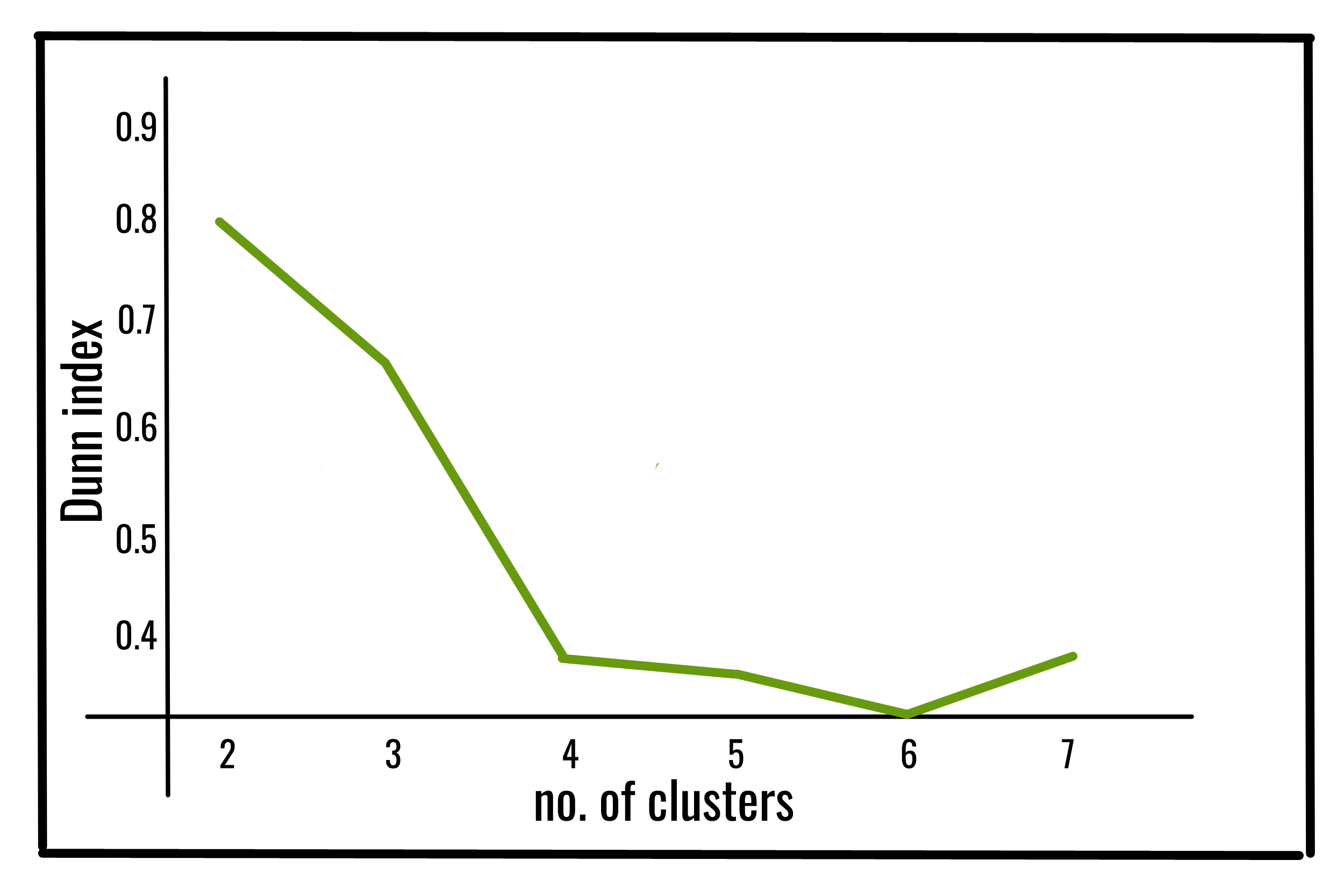Below is the Python implementation of above Dunn index using the jqmcvi library :

 `import` `pandas as pd ` `from` `sklearn ``import` `datasets ` `from` `jqmcvi ``import` `base ` ` `  `# loading the dataset ` `X ``=` `datasets.load_iris() ` `df ``=` `pd.DataFrame(X.data) ` ` `  `# K-Means ` `from` `sklearn ``import` `cluster ` `k_means ``=` `cluster.KMeans(n_clusters``=``3``) ` `k_means.fit(df) ``#K-means training ` `y_pred ``=` `k_means.predict(df) ` ` `  `# We store the K-means results in a dataframe ` `pred ``=` `pd.DataFrame(y_pred) ` `pred.columns ``=` `[``'Type'``] ` ` `  `# we merge this dataframe with df ` `prediction ``=` `pd.concat([df, pred], axis ``=` `1``) ` ` `  `# We store the clusters ` `clus0 ``=` `prediction.loc[prediction.Species ``=``=` `0``] ` `clus1 ``=` `prediction.loc[prediction.Species ``=``=` `1``] ` `clus2 ``=` `prediction.loc[prediction.Species ``=``=` `2``] ` `cluster_list ``=` `[clus0.values, clus1.values, clus2.values] ` ` `  `print``(base.dunn(cluster_list)) `

Output:

```0.67328051
```

### DB index :

The Davies–Bouldin index (DBI) (introduced by David L. Davies and Donald W. Bouldin in 1979), a metric for evaluating clustering algorithms, is an internal evaluation scheme, where the validation of how well the clustering has been done is made using quantities and features inherent to the dataset.
Lower the DB index value, better is the clustering. It also has a drawback. A good value reported by this method does not imply the best information retrieval.

The DB index for k number of clusters is defined as :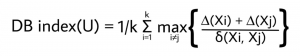where,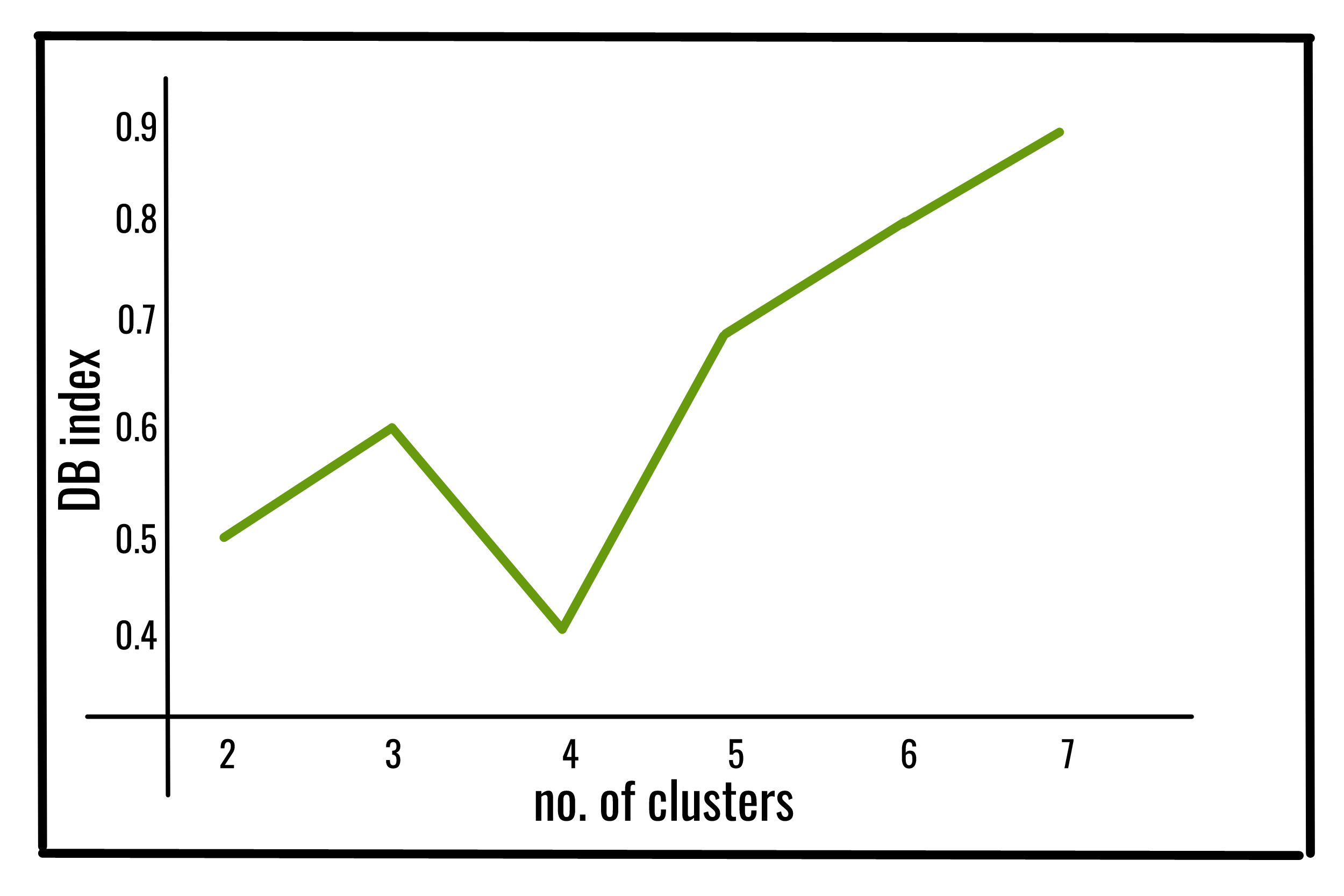Below is the Python implementation of above DB index using the sklearn library :

 `from` `sklearn ``import` `datasets ` `from` `sklearn.cluster ``import` `KMeans ` `from` `sklearn.metrics ``import` `davies_bouldin_score ` `from` `sklearn.datasets.samples_generator ``import` `make_blobs ` ` `  `# loading the dataset ` `X, y_true ``=` `make_blobs(n_samples``=``300``, centers``=``4``,  ` `                       ``cluster_std``=``0.50``, random_state``=``0``) ` ` `  `# K-Means ` `kmeans ``=` `KMeans(n_clusters``=``4``, random_state``=``1``).fit(X) ` ` `  `# we store the cluster labels ` `labels ``=` `kmeans.labels_ ` ` `  `print``(davies_bouldin_score(X, labels)) `

Output:

```0.36628770
```

My Personal Notes arrow_drop_upCheck out this Author's contributed articles.

If you like GeeksforGeeks and would like to contribute, you can also write an article using contribute.geeksforgeeks.org or mail your article to contribute@geeksforgeeks.org. See your article appearing on the GeeksforGeeks main page and help other Geeks.

Please Improve this article if you find anything incorrect by clicking on the "Improve Article" button below.

Article Tags :
Practice Tags :

1

Please write to us at contribute@geeksforgeeks.org to report any issue with the above content.